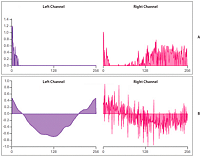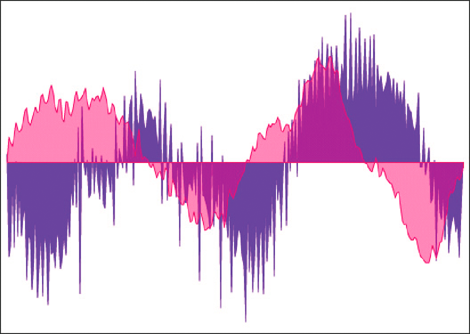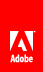Accessing raw sound data

Flash Player 9 and later, Adobe AIR 1.0 and later

The SoundMixer.computeSpectrum() method lets an application read the raw sound data for the waveform that is currently being played. If more than one SoundChannel object is currently playing the SoundMixer.computeSpectrum() method shows the combined sound data of every SoundChannel object mixed together.

The sound data is returned as a ByteArray object containing 512 bytes of data, each of which contains a floating point value between -1 and 1. These values represent the amplitude of the points in the sound waveform being played. The values are delivered in two groups of 256, the first group for the left stereo channel and the second group for the right stereo channel.

The SoundMixer.computeSpectrum() method returns frequency spectrum data rather than waveform data if the FFTMode parameter is set to true . The frequency spectrum shows amplitude arranged by sound frequency, from lowest frequency to highest. A Fast Fourier Transform (FFT) is used to convert the waveform data into frequency spectrum data. The resulting frequency spectrum values range from 0 to roughly 1.414 (the square root of 2).

The following diagram compares the data returned from the computeSpectrum() method when the FFTMode parameter is set to true and when it is set to false . The sound whose data was used for this diagram contains a loud bass sound in the left channel and a drum hit sound in the right channel.
View full size graphicValues returned by the SoundMixer.computeSpectrum() method
A.
fftMode=true

B.
fftMode=false

The computeSpectrum() method can also return data that has been resampled at a lower bit rate. Generally, this results in smoother waveform data or frequency data at the expense of detail. The stretchFactor parameter controls the rate at which the computeSpectrum() method data is sampled. When the stretchFactor parameter is set to 0, the default, the sound data is sampled at a rate of 44.1 kHz. The rate is halved at each successive value of the stretchFactor parameter, so a value of 1 specifies a rate of 22.05 kHz, a value of 2 specifies a rate of 11.025 kHz, and so on. The computeSpectrum() method still returns 256 bytes per stereo channel when a higher stretchFactor value is used.

The SoundMixer.computeSpectrum() method has some limitations:

• Because sound data from a microphone or from RTMP streams do not pass through the global SoundMixer object, the SoundMixer.computeSpectrum() method will not return data from those sources.

• If one or more of the sounds being played come from sources outside the current content sandbox, security restrictions will cause the SoundMixer.computeSpectrum() method to throw an error. For more detail about the security limitations of the SoundMixer.computeSpectrum() method please see Security considerations when loading and playing sounds and Accessing loaded media as data .

However, in an AIR application, content in the application security sandbox (content installed with the AIR application) are not restricted by these security limitations.

Building a simple sound visualizer

The following example uses the SoundMixer.computeSpectrum() method to show a chart of the sound waveform that animates with each frame:

import flash.display.Graphics;
import flash.events.Event;
import flash.media.Sound;
import flash.media.SoundChannel;
import flash.media.SoundMixer;
import flash.net.URLRequest;

const PLOT_HEIGHT:int = 200;
const CHANNEL_LENGTH:int = 256;

var snd:Sound = new Sound();
var req:URLRequest = new URLRequest("bigSound.mp3");

var channel:SoundChannel;
channel = snd.play();

var bytes:ByteArray = new ByteArray();

function onEnterFrame(event:Event):void
{
SoundMixer.computeSpectrum(bytes, false, 0);

var g:Graphics = this.graphics;

g.clear();
g.lineStyle(0, 0x6600CC);
g.beginFill(0x6600CC);
g.moveTo(0, PLOT_HEIGHT);

var n:Number = 0;

// left channel
for (var i:int = 0; i < CHANNEL_LENGTH; i++)
{
n = (bytes.readFloat() * PLOT_HEIGHT);
g.lineTo(i * 2, PLOT_HEIGHT - n);
}
g.lineTo(CHANNEL_LENGTH * 2, PLOT_HEIGHT);
g.endFill();

// right channel
g.lineStyle(0, 0xCC0066);
g.beginFill(0xCC0066, 0.5);
g.moveTo(CHANNEL_LENGTH * 2, PLOT_HEIGHT);

for (i = CHANNEL_LENGTH; i > 0; i--)
{
n = (bytes.readFloat() * PLOT_HEIGHT);
g.lineTo(i * 2, PLOT_HEIGHT - n);
}
g.lineTo(0, PLOT_HEIGHT);
g.endFill();
}

function onPlaybackComplete(event:Event)
{
removeEventListener(Event.ENTER_FRAME, onEnterFrame);
}

This example first loads and plays a sound file and then listens for the Event.ENTER_FRAME event which will trigger the onEnterFrame() method while the sound plays. The onEnterFrame() method starts by calling the SoundMixer.computeSpectrum() method, which stores the sound wave data in the bytes ByteArray object.

The sound waveform is plotted using the vector drawing API. A for loop cycles through the first 256 data values, representing the left stereo channel, and draws a line from each point to the next using the Graphics.lineTo() method. A second for loop cycles through the next set of 256 values, plotting them in reverse order this time, from right to left. The resulting waveform plots can produce an interesting mirror-image effect, as shown in the following image.// Ethnio survey code removed